# SVM理解

## 先说说点到直线的距离

$ax+by+c=0$ ，我们一般的理解是$y=kx+b$，两种形式一样的，实际上是 $y=\frac{a}{b}x+\frac{c}{b}$。 一个点$(x_0,y_0)$到直线的距离D是怎样的呢？ 是D=$\frac{|ax_0+by_0+c|}{\sqrt{a^2+b^2}}$，这个其实一般人都不讲，假设默认知道，其实，推导还挺复杂的，网上有点到直线距离的7种推导,茴香豆的7种写法，呵呵，不过感兴趣的同学可以去研究一下。

$b$是个标量哈，$w$是向量，$x$是向量，别晕哈。### SVM基础目标函数I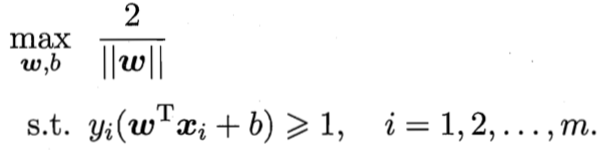### SVM拉格朗日函数

$d^* = g(\lambda^* ,\nu^* )$

$\leq f_0(x^* ) + \sum \lambda_i^* f_i(x^*) + \sum \nu_i^* h_i( x^* )$

$= f_0(x^* )+\sum \lambda_i^* f_i(x^* )$

$\leq f_0(x^* )$

$=p^*$

[参考] 说说g函数。我的理解是，他是一个关于$\lambda, \nu$的函数，啥意思，就是你取值某个$\lambda, \nu$，就会得到一个值，这叫函数呀。那这个值怎么来的呢？是拉格朗日函数的在x取值范围内的下确界，讲人话，就是你给定一个$\lambda_0, \nu_0$，我就去算整个拉格朗日L函数的下确界，就是最小值，这个值就是g的值；然后你再给定一个$\lambda_1, \nu_1$，我又去得到一个关于x作为自变量下拉格朗日L函数的最小值；。。。。；这样下来，就形成了一个$\lambda, \nu$到L最小值的映射关系，这个映射关系，就是g函数。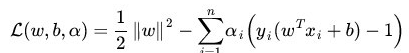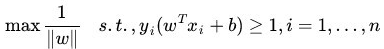### 然后是推导

$L(w,b,\alpha)=\frac{1}{2} ||w|| ^2 + \sum_{i=1}^n \alpha_i (1 - y_i(w^T x_i + b))$ $L(w,b,\alpha)=\frac{1}{2} ||w|| ^2 - \sum_{i=1}^n \alpha_i y_i(w^T x_i + b) + \sum_{i=1}^n \alpha_i$

$\nabla_wL(w,b,\alpha)=w - \sum_{i=1}^n \alpha_i y_i x_i = 0$ $\nabla_bL(w,b,\alpha)= - \sum_{i=1}^n \alpha_i y_i = 0$

$w = \sum_{i=1}^n \alpha_i y_i x_i$ $0 = \sum_{i=1}^n \alpha_i y_i$

$L(w,b,\alpha)=-\frac{1}{2} \sum_{i=1}^n \sum_{j=1}^n \alpha_i \alpha_i y_i y_j x_i x_j + \sum_{i=1}^n \alpha_i$

### 松弛因子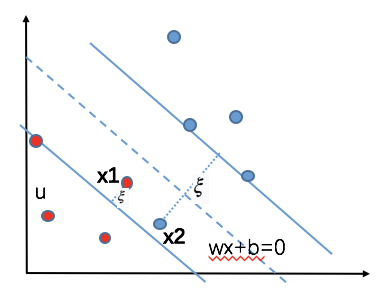C是个超参数，相当于在算每个点算分割超平面的距离的时候，不再是用$\xi$了,而是$C\cdot \xi$，这里我想吐槽一下，可能是我真的没明白，我的理解，这里距离上用$C\cdot \xi$了，那约束上是不是也应该用$C\cdot \xi$，就像： s.t.$y_i(w^T \cdot x_i + b) \geq 1- C \cdot \xi$， 这样我们就好理解多了，为何一个加C，一个不加C呢？

anyway，有了这个超参，我们就可以控制这个越界的个数了，尽量让。 ？？？？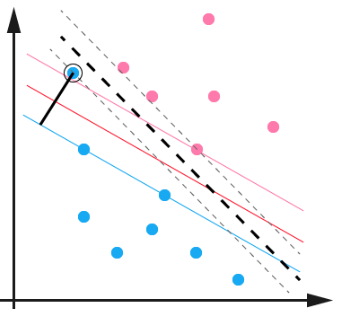### 核函数

Mercer条件，噢，没能力再钻进去思考了，不学数学，我想也就到这步把，让学数学的同学们替我们保证好吧。## 参考

https://blog.csdn.net/v_july_v/article/details/7624837

## 更新

【2019/2/22 更新】

SVM就是这么一个思路，难点是距离的理解，本质是为了简化形式；然后是拉格朗日+对偶的折腾，最大最小换来换去，目的是处理好约束下的最优化；然后是SMO理解一下，目的是为了求解那一堆的$\alpha$；然后核函数,目的是为了减少内积计算；最后是松弛因子，目的是引入一个弹性因子来让距离可以变负，容忍一些节点的位置，从而让模型泛化能力更强。